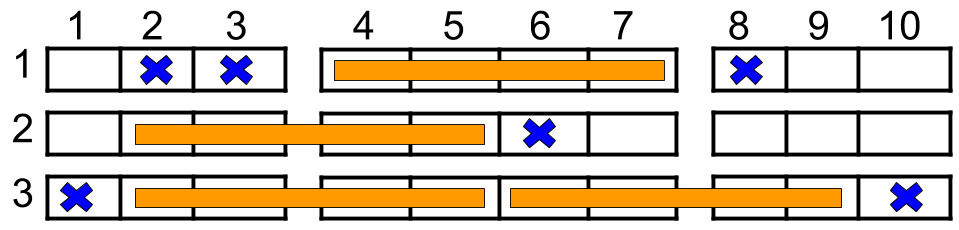##### Welcome to Subscribe On Youtube

Formatted question description: https://leetcode.ca/all/1386.html

# 1386. Cinema Seat Allocation (Medium)A cinema has n rows of seats, numbered from 1 to n and there are ten seats in each row, labelled from 1 to 10 as shown in the figure above.

Given the array reservedSeats containing the numbers of seats already reserved, for example, reservedSeats[i]=[3,8] means the seat located in row 3 and labelled with 8 is already reserved.

Return the maximum number of four-person families you can allocate on the cinema seats. A four-person family occupies fours seats in one row, that are next to each other. Seats across an aisle (such as [3,3] and [3,4]) are not considered to be next to each other, however, It is permissible for the four-person family to be separated by an aisle, but in that case, exactly two people have to sit on each side of the aisle.

Example 1:Input: n = 3, reservedSeats = [[1,2],[1,3],[1,8],[2,6],[3,1],[3,10]]
Output: 4
Explanation: The figure above shows the optimal allocation for four families, where seats mark with blue are already reserved and contiguous seats mark with orange are for one family.


Example 2:

Input: n = 2, reservedSeats = [[2,1],[1,8],[2,6]]
Output: 2


Example 3:

Input: n = 4, reservedSeats = [[4,3],[1,4],[4,6],[1,7]]
Output: 4


Constraints:

• 1 <= n <= 10^9
• 1 <= reservedSeats.length <= min(10*n, 10^4)
• reservedSeats[i].length == 2
• 1 <= reservedSeats[i] <= n
• 1 <= reservedSeats[i] <= 10
• All reservedSeats[i] are distinct.

Related Topics:
Array, Greedy

## Solution 1.

• class Solution {
public int maxNumberOfFamilies(int n, int[][] reservedSeats) {
Arrays.sort(reservedSeats, new Comparator<int[]>() {
public int compare(int[] reservedSeat1, int[] reservedSeat2) {
return reservedSeat1 - reservedSeat2;
}
});
int families = 0;
int prevRow = 0;
Set<Integer> set = new HashSet<Integer>();
int length = reservedSeats.length;
for (int i = 0; i < length; i++) {
int[] reservedSeat = reservedSeats[i];
int row = reservedSeat, column = reservedSeat;
if (column == 1 || column == 10)
continue;
if (row == prevRow)
else {
if (prevRow > 0) {
families += maxNumberOfFamilies(set);
set.clear();
}
for (int j = prevRow + 1; j < row; j++)
families += 2;
prevRow = row;
}
}
families += maxNumberOfFamilies(set);
for (int j = prevRow + 1; j <= n; j++)
families += 2;
return families;
}

public int maxNumberOfFamilies(Set<Integer> set) {
if (set.isEmpty())
return 2;
boolean flag1 = set.contains(4) || set.contains(5);
boolean flag2 = set.contains(6) || set.contains(7);
if (flag1 && flag2)
return 0;
else if (!flag1 && !flag2)
return 1;
else if (flag1) {
if (!set.contains(8) && !set.contains(9))
return 1;
else
return 0;
} else {
if (!set.contains(2) && !set.contains(3))
return 1;
else
return 0;
}
}
}

############

class Solution {
public int maxNumberOfFamilies(int n, int[][] reservedSeats) {
Map<Integer, Integer> m = new HashMap<>();
for (int[] e : reservedSeats) {
int i = e, j = 10 - e;
int v = m.getOrDefault(i, 0);
v |= 1 << j;
m.put(i, v);
}
int[] masks = {0b0111100000, 0b0000011110, 0b0001111000};
int ans = (n - m.size()) << 1;
for (int v : m.values()) {
if ((v & mask) == 0) {
++ans;
}
}
}
return ans;
}
}

• // OJ: https://leetcode.com/problems/cinema-seat-allocation/
// Time: O(SlogS)
// Space: O(1)
class Solution {
public:
int maxNumberOfFamilies(int n, vector<vector<int>>& A) {
sort(A.begin(), A.end());
int ans = 2 * n, i = 0, N = A.size();
while (i < N) {
vector<int> v(4,1);
int j = i;
for (;j < N && A[i] == A[j];++j) {
if (A[j] == 1 || A[j] == 10) continue;
v[A[j]/2-1] = 0;
}
int c = 0;
if (v && v) ++c;
if (v && v) ++c;
if (!c && v && v) ++c;
i = j;
ans -= 2 - c;
}
return ans;
}
};

• class Solution:
def maxNumberOfFamilies(self, n: int, reservedSeats: List[List[int]]) -> int:
m = defaultdict(int)
for i, j in reservedSeats:
m[i] = m[i] | (1 << (10 - j))
ans = (n - len(m)) << 1
for v in m.values():
if (v & mask) == 0:
ans += 1
return ans


• func maxNumberOfFamilies(n int, reservedSeats [][]int) int {
m := map[int]int{}
for _, e := range reservedSeats {
i, j := e, 10-e
m[i] |= 1 << j
}
ans := (n - len(m)) << 1
for _, v := range m {
if (v & mask) == 0 {
ans++
}
}
}
return ans
}

• function maxNumberOfFamilies(n: number, reservedSeats: number[][]): number {
const d: Map<number, number> = new Map();
for (const [i, j] of reservedSeats) {
d.set(i, (d.get(i) ?? 0) | (1 << (10 - j)));
}
let ans = (n - d.size) << 1;
const masks = [0b0111100000, 0b0000011110, 0b0001111000];
for (let [_, x] of d) {
if ((x & mask) === 0) {